Courses

# NCERT Solutions(Part - 2) - Integers Class 7 Notes | EduRev

## Class 7 : NCERT Solutions(Part - 2) - Integers Class 7 Notes | EduRev

The document NCERT Solutions(Part - 2) - Integers Class 7 Notes | EduRev is a part of the Class 7 Course Mathematics (Maths) Class 7.
All you need of Class 7 at this link: Class 7

Exercise 1.2
Ques 1: Write down a pair of integers whose:
(a) sum is -7
(b) difference is -10
(c) sum is 0

Ans:
(a) One such pair whose sum is -7:  -5 + (-2) = -7
(b) One such pair whose difference is -10:  -2 - (+8) = -10
(c) One such pair whose sum is 0:  -5 + 5 = 0

Ques 2: (a) Write a pair of negative integers whose difference gives 8.
(b) Write a negative integer and a positive integer whose sum is -5.
(c) Write a negative integer and a positive integer whose difference is -3.
Ans:
(a) (-2) - (-10) = - 2 + 10 = 8
(b) (-7)+2 = -5
(c) (-2) - 1 = - 2 - 1 = -3

Ques 3: In a quiz, team A scored -40,10,0 and team B scores 10, 0, -40 in three successive rounds. Which team scored more? Can we say that we can add integers in any order?

Ans:
Team A scored -40,10,0
⇒ Total score of Team A = -40 + 10 + 0 = - 30
Team B scored 10,0,-40
⇒ Total score of Team B = 10 + 0+ (-40) - 10 + 0 - 40 = -30
Thus, scores of both teams are same.
Yes, we can add integers in any order due to commutative property.

Ques 4: Fill in the blanks to make the following statements true:
(i) (-5)+(8) = (-8) + (....)
(ii) -53 +... = -53
(iii) 17 +... = 0
(iv) [13 + (-12)] + (....) = 13 + [(-12) + (-7)]
(v)  (-4) + [15 + (-3)] = [-4 + 15] + .....
Ans:
(i) (-5) + (-8) = (-8) + (-5)  [Commutative property]
(ii) -53 + 0 = -53  [Zero additive property]
(iii) 17 + (-17) = 0  (Additive identity]
(iv) [13 + (12)] + (-7) = 13 + [(-12) + (-7)]  [Associative property]
(v) (-4) + [5 + (-3)] = [-4 + 15] + (-3)  [Associative property]

Exercise 1.3
Ques 1:  Find the each of the following products:
(a) 3 x (-1)
(b) (-1) x 225
(c) (-21) x (-30)
(d) (-316) x (-1)
(e) (-15) x 0 x (-18)
(f) (-12) x (-11) x (10)
(g) 9 x (-3) x (-6)
(h) (-18) x (-5) x (-4)
(i) (-1) x (-2) x (-3) x 4
(j) (-3) x (-6) x (2) x (-1)
Ans:
(a) 3 x (-1) = -3
(b) (-1) x 225 = -225
(c) (-21) x (-30) =630
(d) (-316) x (-1) =316
(e) (-15) x 0 x (-18) = 0
(f) (-12) x (-11) x (10) = 132 x 10 = 1320
(g) 9 x (-3) x (-6) = 9 x 18= 162
(h) (-18) x (-5) x (-4) = 90 x (-4) = -360
(i) (-1) x (-2) x (-3) x 4 = (-6 x 4) = -24
(j) (-3) x (-6) x (2) x (-1) = (-18) x (-2) = 36

Ques 2: Verify the following:
(a) 18 x [7 + (-3)] = [18 x 7] + [18 x (-3)]
(b)(-21) x [(-4) + (-6)] = [(-21) x (-4)] + [(-21) x (-6)]
Ans: (a) 18 x [7 + (-3)] = [18 x 7] + [18 x (-3)]
⇒ 18 x 4 = 126 + (-54)
⇒ 72 = 72
⇒ L.H.S. = R.H.S.
Hence, Verified.

(b) (-21) x [(-4) + (-6)] = [(-21)x (-4)] + [(-21) x (-6)]
⇒ (-21) x (-10) = 84 + 126
⇒ 210 = 210
⇒ L.H.S. = R.H.S.
Hence, Verified.

Ques 3: (i) For any integer a, what is (-1) x a equal to?
(ii) Determine the integer whose product with (-1) is:
(a) -22
(b) 37
(c) 0
Ans: (i) (-1) x a = -a, where a is an integer
(ii) (a) (-1) x (-22) = 22
(b) (-1) x 37 =-37
(c) (-1) x 0 = 0

Ques 4: Starting from (-1) x 5, write various products showing some patterns to show (-1) x (-1) = 1
Ans: According to the pattern,

(−1)×(5)=−5
(−1)×(4)=−4
(−1)×(3)=−3
(−1)×(2)=−2
(−1)×(1)=−1
(−1)×(0)=0
(−1)×(−1)=1
Thus, we can conclude that this pattern shows the product of one negative integer and one positive integer is negative integer whereas the product of two negative integers is a positive integer.

Ques 5: Find the product, using suitable properties:
(a) 26 x (-48) +(-48) x (-36)
(b) 8 x 53 x (-125)
(c) 15 x (-25) x (-4) x (-10)
(d) (-41) x (102)
(e) 625 x (-35) + (-625) x 65
(f) 7 x (50-2)
(g) (-7) x (-29)
(h)  (-57) x (-19)+57

Ans:
(a) 26 x (-48) + (-48) x (-36)
⇒ (-48) x [26 + (-36)] [Distributive property]
⇒  (-48) x (-10)
⇒  480

(b) 8 x 53 x (-125)
⇒ 53 x [8 x (-125)] [Commutative property]
⇒  53 x (-1000)
⇒  -53000

(c) 15 x (-25) x (-4) x (-10)
⇒  15 x [(-25) x (-4) x (-10)]  [Commutative property]
⇒ 15  x (-1000)
⇒  -15000

(d) (-41) x (102)
⇒ -41 x [100+2]  [Distributive property]
⇒ [(-41) x 100]+[(-41) x 2]
⇒ -4100 + (-82)
⇒  -4182

(e) 625 x (-35)+(-625) x 65
⇒ 625 x [(-35) + (-65)] [Distributive property]
⇒ 625 x (-100)
⇒ -62500

(f) 7 x (50-2)
⇒ 7 x 50 - 7 x 2    [Distributive property]
⇒ 350-14 = 336

(g)  (17) x (-29)
⇒  (-17) x [(-30) + 1]    [Distributive property]
⇒ (-17) x (30) + (- 17)x 1
⇒   510 + (-17)
⇒  493

(h) (-57) x (-19) + 57
⇒ (-57) x (-19) + 57 x 1
⇒  57 x 19 + 57 x 1
⇒  57 x (19+1)    [Distributive property]
⇒  57 x 20 = 1140

Ques 6: A certain freezing process requires that room temperature be lowered from 40oC at the rate of 5oC every hour. What will be the room temperature 10 hours after the process begins?
Ans: Given: Present room temperature = 40°C
Decreasing the temperature every hour = 5°C
Room temperature after 10 hours = 40°C + 10 x (-5°C)
= 40°C - 50°C
= - 10°C
Thus, the room temperature after 10 hours is - 10°C after the process begins.

Ques 7: In a class test containing 10 questions, 5 marks are awarded for every correct answer and (-2) marks are awarded for every incorrect answer and 0 for questions not attempted.
(i) Mohan gets four correct and six incorrect answers. What is his score?
(ii) Reshma gets five correct answers and five incorrect answers, what is her score?
(iii) Heena gets two correct and five incorrect answers out of seven questions she attempts. What is her score?
Ans:
(i) Mohan gets marks for four correct questions = 4 x 5 = 20
He gets marks for six incorrect questions = 6 x (-2) = -12
Therefore, total scores of Mohan = (4 x 5) + [6 x (-2)]
= 20- 12 = 8
Thus, Mohan gets 8 marks in a class test

(ii) Reshma gets marks for five correct questions = 5 x 5 = 25
She gets marks for five incorrect questions = 5 x (-2)=-10
Therefore, total score of Resham = 25 + (-10) = 15
Thus, Reshma gets 15 marks in a class test

(iii)  Heena gets marks for two correct questions = 2 x 5 = 10
She gets marks for five incorrect questions = 5 x (-2) = -10
Therefore, total score of Resham = 10 + (-10) = 0
Thus, Reshma gets 0 marks in a class test

Ques 8: A cement company earns a profit of Rs 8 per bag of white cement sold and a loss of Rs 5 per bag of grey cement sold.
(a) The company sells 3,000 bags of white cement and 5,000 bags of grey cement in a month. What is its profit or loss?
(b) What is the number of white cement bags it must sell to have neither profit nor loss. If the number of grey bags sold is 6,400 bags.
Ans: Given: Profit of 1 bag of white cement = Rs 8
And Loss of 1 bag of grey cement = Rs 5
(a) Profit on selling 3000 bags of white cement = 3000 x Rs 8 = Rs 24,000
Loss of selling 5000 bags of grey cement = 5000 x Rs 5 = Rs 25,000
Since, Profit < Loss
Therefore, his total loss on selling the grey cement bags = Loss - Profit
= Rs 25,000 - Rs 24,000
= Rs 1,000
Thus, he has lost of Rs 1,000 on selling the grey cement bags.

(b) Let the number of bags of white cement be x.
According to question,Thus, he must sell 4000 white cement bags to have neither profit nor loss.

Ques 9: Replace the blank with an integer to make it a true statement:
(a) (-3) x _____= 27
(b) 5 x _____    = -35
(c)  _____ x (-8) = -56
(d) _____  x (-12) = 132
Ans: (a) (-3) x (-9) = 27
(b) 5 x (-7) = -35
(c) 7 x (-8) = -56
(d) (-11) x (-12) = 132

Exercise 1.4
Ques 1: Evaluate each of the following:
(a) (-30) ÷ 10
(b) 50 ÷ (-5)
(c) (-36) ÷ (-9)
(d) (-49) ÷ 49
(e) 13 + [(-2) + 1]
(f) 0 ÷ (-12)
(g) (-31) ÷ [(-30) ÷(-1)]
(h) [(-36) ÷ 12] ÷ 3
(i) [(-6)+5] ÷ [(-2) + 1]
Ans: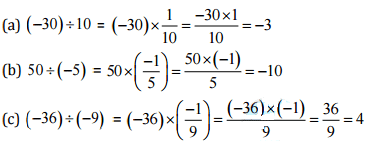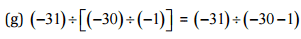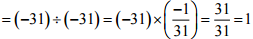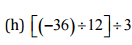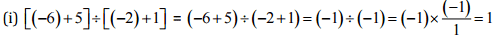Ques 2: Verify that a+(b + c)≠(a+b)+(a ÷ c) for each of the following values of a,b and c.
(a) a = 12, b = -4,c = 2
(b) a = (-10), b = 1 c = 1
Ans:
(a) Given: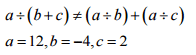Putting the given values in L.H.S,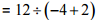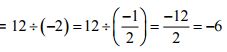Putting the given values in L.H.S.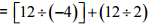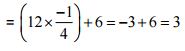Since, L.H.S. ≠ R.H.S. Hence verified.
Putting the given values in L.H.S, = 12 +(-4 +2)

(b) Given:   a÷(b+c)≠(a÷b) + (a÷c)
a = -10,b=1,c=1
Putting the given values in L.H.S. = -10 ÷ (l + 1)
= -10 ÷ (2) = -5
Putting the given values in R.H.S. = [-10÷1]+ (-10÷ 1)
= -10 -10 = -20
Since,  L.H.S. ≠ R.H.S.
Hence verified.

Ques 3: Fill in the blanks:
(a) 369 ÷ _____   = 369
(b) (-75) ÷ _____   = (-1)
(c) (-206) ÷ _____   = 1
(d) (-87) ÷  _____  = 87
(e)  _____  ÷1 = -87
(f)  _____  ÷ 48 = -1
(g) 20 ÷  _____  = -2
(h) _____   ÷ (4) = -3
Ans:
(a) 369 ÷ 1 = 369
(b) (-75) ÷ 75 = (-1)
(c) (-206) ÷ (-206) = 1
(d) (-87) ÷ (-1) = 87
(e) (-87)÷ = -87
(f) (-48) ÷ 48 = -1
(g) 20 ÷ (-10) = -2
(h) (-12) ÷ (4) = -3

Ques 4: Write five pairs of integers (a,b) such that a ÷ b = -3. One such pair is (6,-2) because 6÷(-2) = (-3)
Ans: (i)  (-6) ÷ 2 = -3
(ii) 9 ÷ (-3) = -3
(iii) 12 ÷ (-4) = -3
(iv) (-9) ÷ 3 = -3
(v) (-15) ÷ 5 = -3

Ques 5: The temperature at noon was 10oC above zero. If it decreases at the rate of 2oC per hour until mid-night, at what time would the temperature be 8oC below zero? What would be the temperature at mid-night?

Ans:
Following number line is representing the temperature: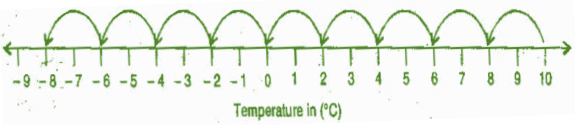The temperature decreases 2oC = 1 hour
The temperature decreases 1°C = 1/2 hour
The temperature decreases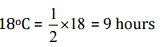Total time = 12 noon + 9 hours = 21 hours = 9 pm
Thus, at 9 pm the temperature would be 8°C below 0°C.

Ques 6: In a class test (+3) marks are given for every correct answer and (-2) marks are given for every incorrect answer and no marks for not attempting any question.
(i) Radhika scored 20 marks. If she has got 12 correct answers, how many questions has she attempted incorrectly?
(ii) Mohini scores (-5) marks in this test, though she has got 7 correct answers.
How many questions has she attempted incorrectly?

Ans: (i) Marks given for one correct answer = 3
Marks given for 12 correct answers = 3 x 12 = 36
Therefore, Marks obtained for incorrect answers = 20 - 36 = -16
Now, marks given for one incorrect answer = -2
Therefore, number of incorrect answers = (-16) ÷(-2) = 8
Thus, Radhika has attempted 8 incorrect questions.

(ii) Marks given for seven correct answers = 3 x 7 = 21
Mohini scores = -5
Marks obtained for incorrect answers = = -5-21 = -26
Now, marks given for one incorrect answer = -2
Therefore, number of incorrect answers = (-26) ÷ (-2) = 13
Thus, Mohini has attempted 13 incorrect questions.

Ques 7: An elevator descends into a mine shaft at the rate of 6 m/min. If the descent starts from 10 above the ground level, how long will it take to reach -350 m?
Ans: Starting position of mine shaft is 10 m above the ground but it moves in opposite direction so it travels the distance (-350) m below the ground.
So total distance covered by mine shaft = 10 m - (-350) m = 10 + 350 = 360 m
Now, time taken to cover a distance of 6 m by it = 1 minute
So, time taken to cover a distance of 1 m by it = 1/6 minute
Therefore, time taken to cover a distance of 360 m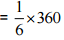= 60 minutes = 1 hour
[Since 60 minutes = 1 hour]
Thus, in one hour the mine shaft reaches -350m below the ground.

Offer running on EduRev: Apply code STAYHOME200 to get INR 200 off on our premium plan EduRev Infinity!

,

,

,

,

,

,

,

,

,

,

,

,

,

,

,

,

,

,

,

,

,

;